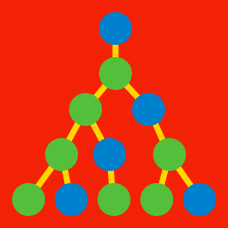Discrete Mathematics

Linear Recurrence Relations: Level 5 Challenges

Let $k_1, k_2, \ldots,$ be a sequence that is recursively defined as $k_{n+2} = k_{n+1} + 2 k_{n}$, for all $n\geq 1$, with $k_1 = k_2 = 1$. The infinite sum, $S = \frac{k_1}{7 ^1} + \frac{k_2}{7 ^2} + \frac{k_3}{7 ^3} \ldots$, is a fraction of the form $\frac{a}{b}$, where $a$ and $b$ are coprime integers. What is the value of $a+b$?

The sequence $a_0,a_1,a_2,\dots$ satisfies $a_{m+n} + a_{m-n} = \frac{1}{2}(a_{2m}+a_{2n})$

for all non-negative integers with $m\geq n$. If $a_1 = 1$, determine the value of $a_{1995}$

Define a subset $S$ of the first $30$ positive integers to be uneven if, for all $i \in S$, $i+2 \notin S$. For example, $\{1, 2\}$ is an uneven subset, while $\{1, 2, 3\}$ is not. If $N$ represents the number of uneven subsets, find the remainder when $N$ is divided by $1000$.

Notes:

The empty set is considered to be an uneven subset.

You may want use a calculator at the end.

{ \begin{aligned} a_{n} & =& 2a_{n-1} + 3 \\ b_{n} &=& b_{n-1} + b_{n-2} \\ \end{aligned} }

For a positive integer $n$, consider the two recurrence relations above subjected to the conditions $a_1 = 0$ and $b_{1} = b_{2} = 1$.

If the value of the expression $\big(a_{b_{2015}} + 3\big)\big(a_{b_{2016}} + 3\big)$ can be expressed as $p^{b_{q}} \frac{r}{s}$, where $b_{q}$ is one of the terms in the recurrence relations sequence above and $(p, r)$ and $(r, s)$ are pairwise coprime integers.

Find the value of $(p+q+r+s) \bmod {673}$.

$\large a_{n+2} = (n + 3)a_{n+1} - (n + 2)a_{n}$

For whole numbers $n$, consider the recurrence relation defined as above with $a_{1} = 1, a_{2} = 3$.

Find $\displaystyle\bigg( \sum_{k=1}^{2015} a_{k} \bigg)\pmod{100}.$

×